## Relativistic Quantities

A velocity-addition formula is an equation that relates the velocities of moving objects in different reference frames.

### Learning Objectives

Express velocity-addition formulas for objects at speeds much less and approaching the speed of light

### Key Takeaways

#### Key Points

• When two objects are moving slowly compared to speed of light, it is accurate enough to use the vector sum of velocities: $\text{s} = \text{u} + \text{v}$.
• As the velocity increases towards the speed of light, the vector sum of velocities is replaced with: $\text{s} = \frac{\text{v} + \text{u}}{1 + \text{vu}/\text{c}^{2}}$.
• Composition law for velocities gave the first test of the kinematics of the special theory of relativity when, using a Michelson interferometer, Hyppolite Fizeau measured the speed of light in a fluid moving parallel to the light.

#### Key Terms

• special relativity: A theory that (neglecting the effects of gravity) reconciles the principle of relativity with the observation that the speed of light is constant in all frames of reference.
• interferometer: Any of several instruments that use the interference of waves to determine wavelengths and wave velocities, determine refractive indices, and measure small distances, temperature changes, stresses, and many other useful measurements.
• speed of light: the speed of electromagnetic radiation in a perfect vacuum: exactly 299,792,458 meters per second by definition

A velocity-addition formula is an equation that relates the velocities of moving objects in different reference frames.

As Galileo Galilei observed in 17th century, if a ship is moving relative to the shore at velocity $\text{v}$, and a fly is moving with velocity $\text{u}$ as measured on the ship, calculating the velocity of the fly as measured on the shore is what is meant by the addition of the velocities $\text{v}$ and $\text{u}$. When both the fly and the ship are moving slowly compared to speed of light, it is accurate enough to use the vector sum $\text{s} = \text{u} + \text{v}$ where $\text{s}$ is the velocity of the fly relative to the shore.

According to the theory of special relativity, the frame of the ship has a different clock rate and distance measure, and the notion of simultaneity in the direction of motion is altered, so the addition law for velocities is changed.

Since special relativity dictates that the speed of light is the same in all frames of reference, light shone from the front of a moving car can’t go faster than light from a stationary lamp. Since this is counter to what Galileo used to add velocities, there needs to be a new velocity addition law.

This change isn’t noticeable at low velocities but as the velocity increases towards the speed of light it becomes important. The addition law is also called a composition law for velocities. For collinear motions, the velocity of the fly relative to the shore is given by the following equation:

$\text{s} = \frac{\text{v} + \text{u}}{1 + \text{vu}/\text{c}^{2}}$.

Composition law for velocities gave the first test of the kinematics of the special theory of relativity. Using a Michelson interferometer, Hyppolite Fizeau measured the speed of light in a fluid moving parallel to the light in 1851. The speed of light in the fluid is slower than the speed of light in vacuum, and it changes if the fluid is moving along with the light. The speed of light in a collinear moving fluid is predicted accurately by the collinear case of the relativistic formula.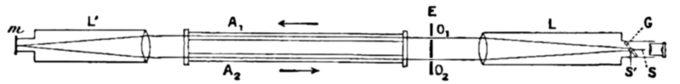Setup of the Fizeau Experiment: A light ray emanating from the source S’ is reflected by a beam splitter G and is collimated into a parallel beam by lens L. After passing the slits O1 and O2, two rays of light travel through the tubes A1 and A2, through which water is streaming back and forth as shown by the arrows. The rays reflect off a mirror m at the focus of lens L’, so that one ray always propagates in the same direction as the water stream, and the other ray opposite to the direction of the water stream. After passing back and forth through the tubes, both rays unite at S, where they produce interference fringes that can be visualized through the illustrated eyepiece. The interference pattern can be analyzed to determine the speed of light traveling along each leg of the tube.

## Relativistic Momentum

Relativistic momentum is given as $\gamma \text{m}_{0}\text{v}$ where $\text{m}_{0}$ is the object’s invariant mass and $\gamma$ is Lorentz transformation.

### Learning Objectives

Compare Newtonian and relativistic momenta for objects at speeds much less and approaching the speed of light

### Key Takeaways

#### Key Points

• Newtonian physics assumes that absolute time and space exist outside of any observer, resulting in a prediction that the speed of light can vary from one reference frame to another.
• In the theory of special relativity, the equations of motion do not depend on the reference frame while the speed of light (c) is invariant.
• Within the domain of classical mechanics, relativistic momentum closely approximates Newtonian momentum. At low velocity $\gamma \text{m}_{0}\text{v}$ is approximately equal to $\text{m}_{0}\text{v}$, the Newtonian expression for momentum.

#### Key Terms

• Galilean transformation: a transformation used to transform between the coordinates of two reference frames which differ only by constant relative motion within the constructs of Newtonian physics.
• Lorentz transformation: a transformation relating the spacetime coordinates of one frame of reference to another in special relativity
• special relativity: A theory that (neglecting the effects of gravity) reconciles the principle of relativity with the observation that the speed of light is constant in all frames of reference.

### Relativistic Momentum

Newtonian physics assumes that absolute time and space exist outside of any observer. This gives rise to Galilean relativity, which states that the laws of motion are the same in all inertial frames. It also results in a prediction that the speed of light can vary from one reference frame to another. However, this is contrary to observation. In the theory of special relativity, Albert Einstein keeps the postulate that the equations of motion do not depend on the reference frame, but assumes that the speed of light c is invariant. As a result, position and time in two reference frames are related by the Lorentz transformation instead of the Galilean transformation.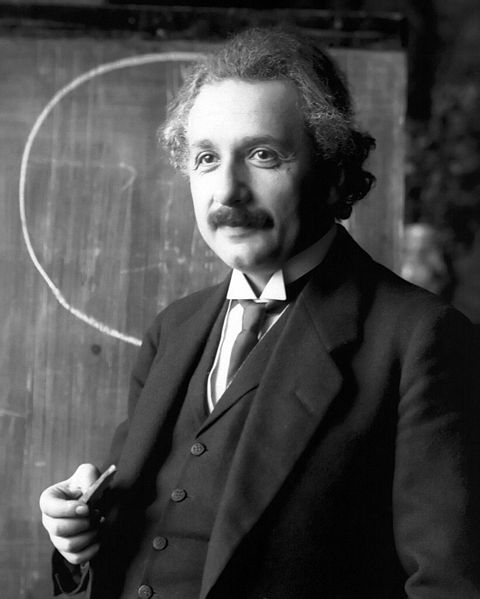Albert Einstein: Albert Einstein in 1921

Consider, for example, a reference frame moving relative to another at velocity v in the x direction. The Galilean transformation gives the coordinates of the moving frame as

$\text{t}' = \text{t}$

$\text{x}' = \text{x} -\text{vt}$

while the Lorentz transformation gives

$\text{t}' = \gamma (\text{t} - \frac{\text{vx}}{\text{c}^{2}})$

$\text{x}' = \gamma (\text{x} - \text{vt})$

where $\gamma$ is the Lorentz factor:

$\gamma = \frac{1}{\sqrt{1 - (\text{v}/\text{c})^{2}}}$.

Conservation laws in physics, such as the law of conservation of momentum, must be invariant. That is, the property that needs to be conserved should remain unchanged regardless of changes in the conditions of measurement. This means that the conservation law needs to hold in any frame of reference. Newton’s second law [with mass fixed in the expression for momentum (p=m*v)], is not invariant under a Lorentz transformation. However, it can be made invariant by making the inertial mass m of an object a function of velocity:

$\text{m} = \gamma \text{m}_{0}$

where $\text{m}_{0}$ is the object’s invariant mass.

The modified momentum,

$\text{p} = \gamma \text{m}_{0}\text{v}$

obeys Newton’s second law:

$\text{F} = \frac{\text{dp}}{\text{dt}}$.

It is important to note that for speeds much less than the speed of light, Newtonian momentum and relativistic momentum are approximately the same. As one approaches the speed of light, however, relativistic momentum becomes infinite while Newtonian momentum continues to increases linearly. Thus, it is necessary to employ the expression for relativistic momentum when one is dealing with speeds near the speed of light.Relativistic and Newtonian Momentum: This figure illustrates that relativistic momentum approaches infinity as the speed of light is approached. Newtonian momentum increases linearly with speed.

## Relativistic Energy and Mass

In special relativity, as the object approaches the speed of light, the object’s energy and momentum increase without bound.

### Learning Objectives

Evaluate possibility for an object to travel at the speed of light

### Key Takeaways

#### Key Points

• In special relativity, an object that has a mass cannot travel at the speed of light.
• Relativistic mass is defined as $\text{m}_{\text{rel}} = \frac{\text{E}}{\text{c}^{2}}$and can be viewed as the proportionality constant between the velocity and the momentum.
• Relativistic energy is connected with rest mass via the following equation: $\text{E}_{\text{r}} = \sqrt{(\text{m}_{0}\text{c}^{2})^{2} + (\text{pc})^{^{2}}}$.

#### Key Terms

• Lorentz factor: The factor, used in special relativity, to calculate the degree of time dilation, length contraction and relativistic mass of an object moving relative to an observer.
• rest mass: the mass of a body when it is not moving relative to an observer
• special relativity: A theory that (neglecting the effects of gravity) reconciles the principle of relativity with the observation that the speed of light is constant in all frames of reference.

### Relativistic Energy and Mass

In special relativity, an object that has a mass cannot travel at the speed of light. As the object approaches the speed of light, the object’s energy and momentum increase without bound. Relativistic corrections for energy and mass need to be made because of the fact that the speed of light in a vacuum is constant in all reference frames. The conservation of mass and energy are well-accepted laws of physics. In order for these laws to hold in all reference frames, special relativity must be applied. It is important to note that for objects with speeds that are well below the speed of light that the expressions for relativistic energy and mass yield values that are approximately equal to their Newtonian counterparts.Relativistic and Newtonian Kinetic Energy: This figure illustrates how relativistic and Newtonian Kinetic Energy are related to the speed of an object. The relativistic kinetic energy increases to infinity when an object approaches the speed of light, this indicates that no body with mass can reach the speed of light. On the other hand, Newtonian kinetic energy continues to increase without bound as the speed of an object increases.

### Relativistic Mass

Relativistic mass was defined by Richard C. Tolman pictured left of Albert Einstein here in 1934 as which holds for all particles, including those moving at the speed of light. For a slower than light particle, a particle with a nonzero rest mass, the formula becomes where is the rest mass and is the Lorentz factor. The Lorentz factor is equal to: $\gamma = \frac{1}{\sqrt{1 - \text{v}^2/\text{c}^2}}$, where v is the relative velocity between inertial reference frames and c is the speed of light.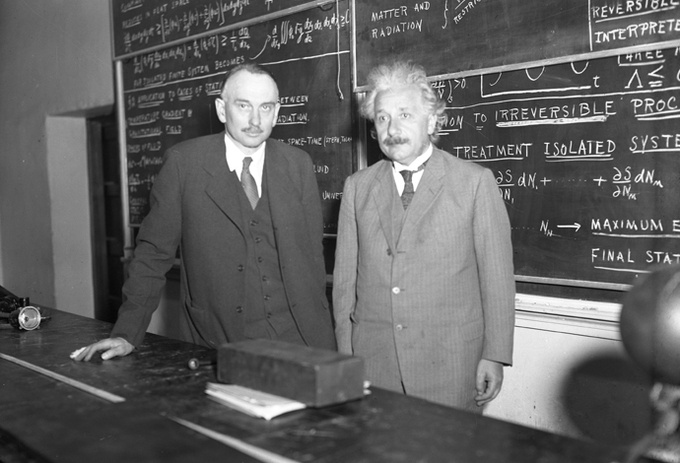Richard C. Tolman and Albert Einstein: Richard C. Tolman (1881 – 1948) with Albert Einstein (1879 – 1955) at Caltech, 1932

When the relative velocity is zero, is simply equal to 1, and the relativistic mass is reduced to the rest mass. As the velocity increases toward the speed of light (c), the denominator of the right side approaches zero, and consequently approaches infinity.

In the formula for momentum the mass that occurs is the relativistic mass. In other words, the relativistic mass is the proportionality constant between the velocity and the momentum.

While Newton’s second law remains valid in the form the derived form is not valid because in is generally not a constant.

### Relativistic Energy

Relativistic energy ($\text{E}_{\text{r}} = \sqrt{(\text{m}_{0}\text{c}^{2})^{2} + (\text{pc})^{^{2}}}$) is connected with rest mass via the following equation: $\text{m} = \frac{\sqrt{(\text{E}^{2} - (\text{pc})^{^{2}}}}{\text{c}^{2}}$. Here the term represents the square of the Euclidean norm (total vector length) of the various momentum vectors in the system, which reduces to the square of the simple momentum magnitude, if only a single particle is considered. This equation reduces to when the momentum term is zero. For photons where the equation reduces to.

Today, the predictions of relativistic energy and mass are routinely confirmed from the experimental data of particle accelerators such as the Relativistic Heavy Ion Collider. The increase of relativistic momentum and energy is not only precisely measured but also necessary to understand the behavior of cyclotrons and synchrotron, which accelerate particles to near the speed of light.

## Matter and Antimatter

Antimatter is composed of antiparticles, which have the same mass as particles of ordinary matter but opposite charge and quantum spin.

### Learning Objectives

Describe properties of antiparticles

### Key Takeaways

#### Key Points

• The end result of antimatter meeting matter is a release of energy proportional to the mass, as shown in the mass-energy equivalence equation, E = mc2.
• Although almost all matter observable from the Earth seems to be made of matter rather than antimatter, antimatter may still exist in relatively large amounts in far-away galaxies.
• No explanation for the apparent asymmetry of matter and antimatter in the visible universe exists.

#### Key Terms

• annihilation: the process of a particle and its corresponding antiparticle combining to produce energy
• positron: The antimatter equivalent of an electron, having the same mass but a positive charge.
• antimatter: matter that is composed of the antiparticles of those that constitute normal matter

Antimatter is material composed of antiparticles, which have the same mass as particles of ordinary matter but have opposite charge and quantum spin. Antiparticles bind with each other to form antimatter in the same way that normal particles bind to form normal matter. For example, a positron (the antiparticle of the electron, with symbol e+) and an antiproton (symbol p) can form an antihydrogen atom. Furthermore, mixing matter and antimatter can lead to the annihilation of both, in the same way that mixing antiparticles and particles does. This gives rise to high-energy photons (gamma rays) and other particle-antiparticle pairs. The end result of antimatter meeting matter is a release of energy proportional to the mass, as shown in the mass-energy equivalence equation, E = mc2.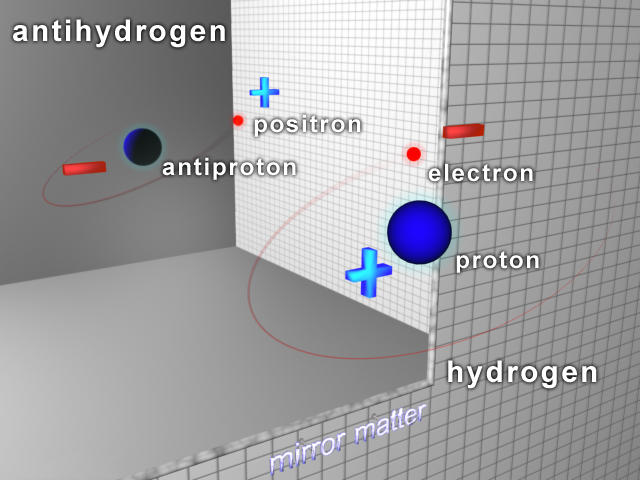Antihydrogen and Hydrogen Atoms: Antihydrogen consists of an antiproton and a positron; hydrogen consists of a proton and an electron.

Almost all matter observable from the earth seems to be made of matter rather than antimatter. If antimatter-dominated regions of space existed, the gamma rays produced in annihilation reactions along the boundary between matter and antimatter regions would be detectable.

Antimatter may still exist in relatively large amounts in far-away galaxies due to cosmic inflation in the primordial time of the universe. Antimatter galaxies, if they exist, are expected to have the same chemistry and absorption and emission spectra as normal-matter galaxies, and their astronomical objects would be observationally identical, making them difficult to distinguish from normal-matter galaxies.

There is considerable speculation as to why the observable universe is apparently composed almost entirely of matter (as opposed to a mixture of matter and antimatter), whether there exist other places that are almost entirely composed of antimatter instead, and what sorts of technology might be possible if antimatter could be harnessed. At this time, the apparent asymmetry of matter and antimatter in the visible universe is one of the greatest unsolved problems in physics.

## Relativistic Kinetic Energy

Relativistic kinetic energy can be expressed as: $\text{E}_{\text{k}} = \frac{\text{mc}^{2}}{\sqrt{1 - (\text{v}/\text{c})^{2})}} - \text{mc}^{2}$ where $\text{m}$ is rest mass, $\text{v}$ is velocity, $\text{c}$ is speed of light.

### Learning Objectives

Compare classical and relativistic kinetic energies for objects at speeds much less and approaching the speed of light

### Key Takeaways

#### Key Points

• Relativistic kinetic energy equation shows that the energy of an object approaches infinity as the velocity approaches the speed of light. Thus it is impossible to accelerate an object across this boundary.
• Kinetic energy calculations lead to the mass -energy equivalence formula: $\text{E}_{\text{rest}} = \text{E}_{0} = \text{mc}^{2}$.
• At a low speed ($\text{v} << \text{c}$), the relativistic kinetic energy may be approximated by the classical kinetic energy. Thus, the total energy can be partitioned into the energy of the rest mass plus the traditional Newtonian kinetic energy at low speeds.

#### Key Terms

• special relativity: A theory that (neglecting the effects of gravity) reconciles the principle of relativity with the observation that the speed of light is constant in all frames of reference.
• classical mechanics: All of the physical laws of nature that account for the behaviour of the normal world, but break down when dealing with the very small (see quantum mechanics) or the very fast or very heavy (see relativity).
• Lorentz factor: The factor, used in special relativity, to calculate the degree of time dilation, length contraction and relativistic mass of an object moving relative to an observer.

In classical mechanics, the kinetic energy of an object depends on the mass of a body as well as its speed. The kinetic energy is equal to the mass multiplied by the square of the speed, multiplied by the constant 1/2. The equation is given as:

$\text{E}_{\text{k}} = \frac{1}{2}\text{mv}^{2}$,

where $\text{m}$ is the mass and $\text{v}$ is the speed (or the velocity) of the body.

The classical kinetic energy of an object is related to its momentum by the equation:

$\text{E}_{\text{k}} = \frac{\text{p}^{2}}{2\text{m}}$,

where $\text{p}$ is momentum.

If the speed of a body is a significant fraction of of the speed of light, it is necessary to employ special relativity to calculate its kinetic energy. It is important to know how to apply special relativity to problems with high speed particles. In special relativity, we must change the expression for linear momentum. Using $\text{m}$ for rest mass, $\text{v}$ and $\nu$ for the object’s velocity and speed respectively, and $\text{c}$ for the speed of light in vacuum, the relativistic expression for linear momentum is: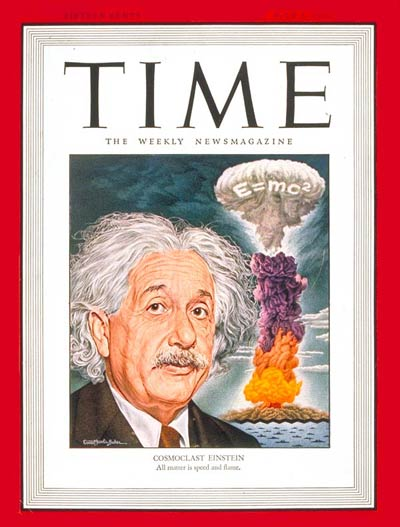Time Magazine – July 1, 1946: The popular connection between Einstein, E = mc2, and the atomic bomb was prominently indicated on the cover of Time magazine (July 1946) by the writing of the equation on the mushroom cloud itself.

$\text{p} = \text{m}\gamma \text{v}$, where $\gamma$ is the Lorentz factor:

$\gamma = \frac{1}{\sqrt{1 - (\text{v}/\text{c})^{2}}}$.

Since the kinetic energy of an object is related to its momentum, we intuitively know that the relativistic expression for kinetic energy will also be different from its classical counterpart. Indeed, the relativistic expression for kinetic energy is:

$\text{E}_{\text{k}} = \frac{\text{mc}^{2}}{\sqrt{1 - (\text{v}/\text{c})^{2})}} - \text{mc}^{2}$.

The equation shows that the energy of an object approaches infinity as the velocity $\text{v}$ approaches the speed of light $\text{c}$. Thus it is impossible to accelerate an object across this boundary.

The mathematical by-product of this calculation is the mass-energy equivalence formula (referred to in ). The body at rest must have energy content equal to:

$\text{E}_{\text{rest}} = \text{E}_{0} = \text{mc}^{2}$.

The general expression for the kinetic energy of an object that is not at rest is:

$\text{KE} = \text{mc}^2-\text{m}_0\text{c}^2$, where m is the relativistic mass of the object and m0 is the rest mass of the object.

At a low speed ($\text{v} << \text{c}$), the relativistic kinetic energy may be approximated well by the classical kinetic energy. We can show this to be true by using a Taylor expansion for the reciprocal square root and keeping first two terms of the relativistic kinetic energy equation. When we do this we get:

$\text{E}_\text{k} \approx \text{m} \text{c}^2 \left(1 + \frac{1}{2} \text{v}^2/\text{c}^2\right) - \text{m} \text{c}^2 = \frac{1}{2} \text{m} \text{v}^2$.

Thus, the total energy can be partitioned into the energy of the rest mass plus the traditional classical kinetic energy at low speeds.